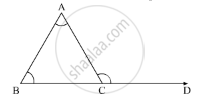Advertisement Remove all ads

# An Exterior Angle of a Triangle is Equal to 100° and Two Interior Opposite Angles Are Equal. Each of These Angles is Equal to - Mathematics

MCQ

An exterior angle of a triangle is equal to 100° and two interior opposite angles are equal. Each of these angles is equal to

#### Options

• 75°

• 80°

• 80°

• 40°

• 50°

Advertisement Remove all ads

#### Solution

n the ΔABCCD is the ray extended from the vertex C of ΔABC. It is given that the exterior angle of the triangle is  100° and two of the interior opposite angles are equal.

So, ∠ACD = 100° and A = ∠BSo, now using the property, “an exterior angle of the triangle is equal to the sum of the two opposite interior angles”, we get.

In ΔABC

∠A + ∠B = ∠ACD

∠2A = 100°

∠A =  (100°)/2

∠A = 50°

∠A = ∠B = 50°

Therefore, each of the two opposite interior angles is 50°.

Is there an error in this question or solution?
Advertisement Remove all ads

#### APPEARS IN

RD Sharma Mathematics for Class 9
Chapter 11 Triangle and its Angles
Q 3 | Page 25
Advertisement Remove all ads
Advertisement Remove all ads
Share
Notifications

View all notifications

Forgot password?
Course